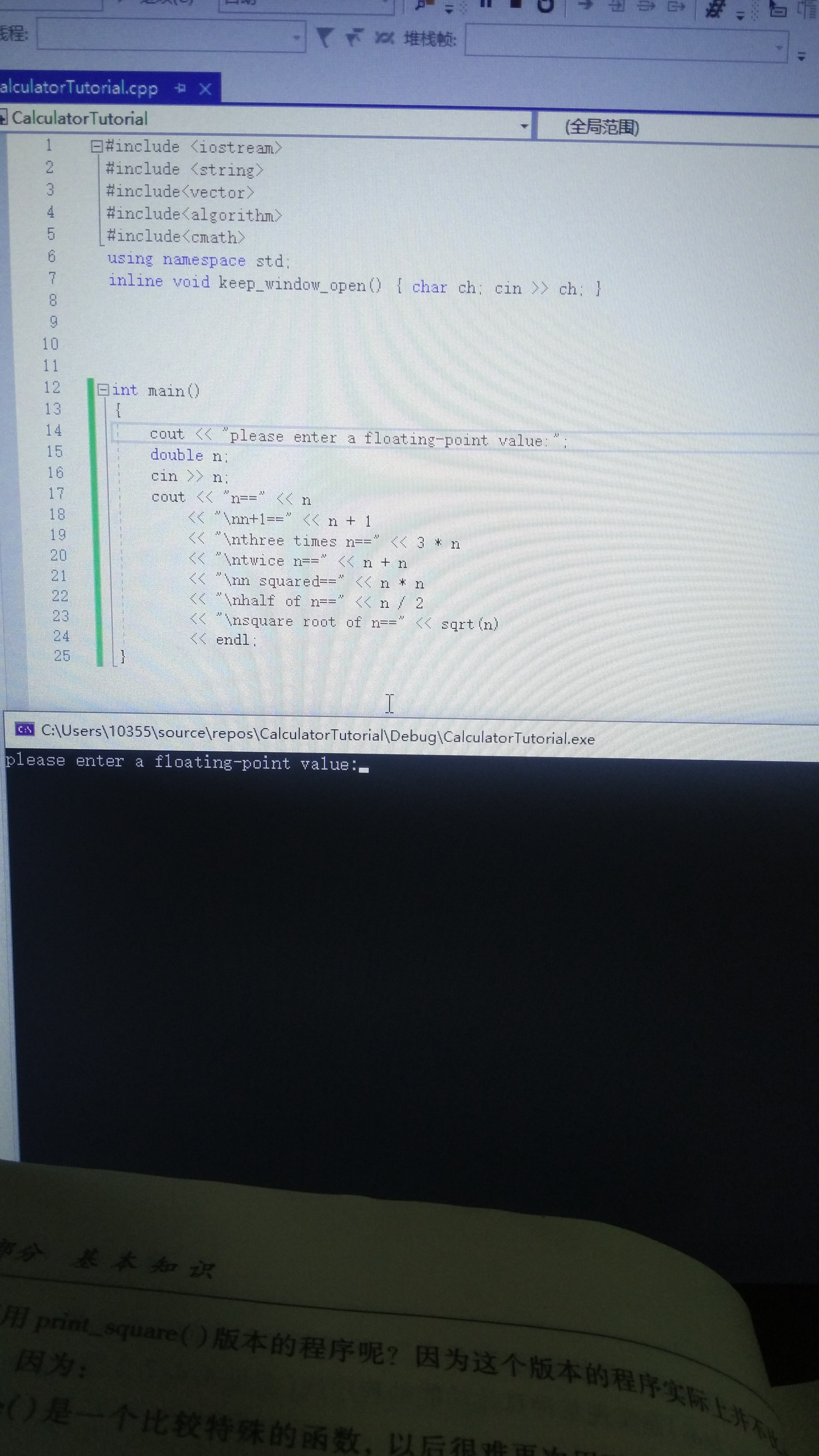Visual Studio C++里面问什么只能输出第一个cout而下面的完全不显示是什么原因呀int main()
{
cout << "please enter a floating-point value:";
double n;
cin >> n;
cout << "n==" << n
<< "\nn+1==" << n + 1
<< "\nthree times n==" << 3 * n
<< "\ntwice n==" << n + n
<< "\nn squared==" << n * n
<< "\nhalf of n==" << n / 2
<< "\nsquare root of n==" << sqrt(n)
<< endl;
}

5个回答clowndong 懂了谢谢
7 个月之前 回复clowndong 请问一下这个输入一个n值具体怎么操作呀 能教一下吗 实在看不懂
7 个月之前 回复

cout 是输出，cin是输入，

cin, cout是标准输入输出流，顾名思义，

``````cout << "please enter a floating-point value:";
``````

``````cin >> n;
``````clowndong 请问一下这个具体怎么操作呀 我实在小白一个 不太懂
7 个月之前 回复